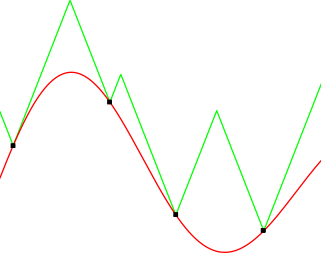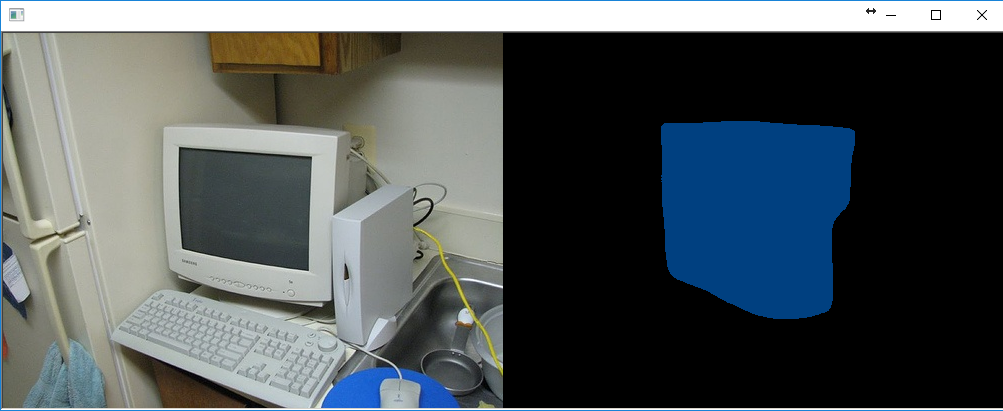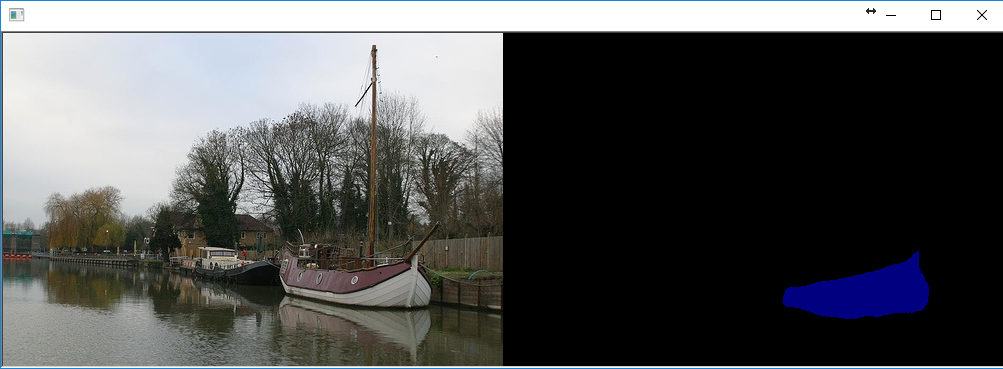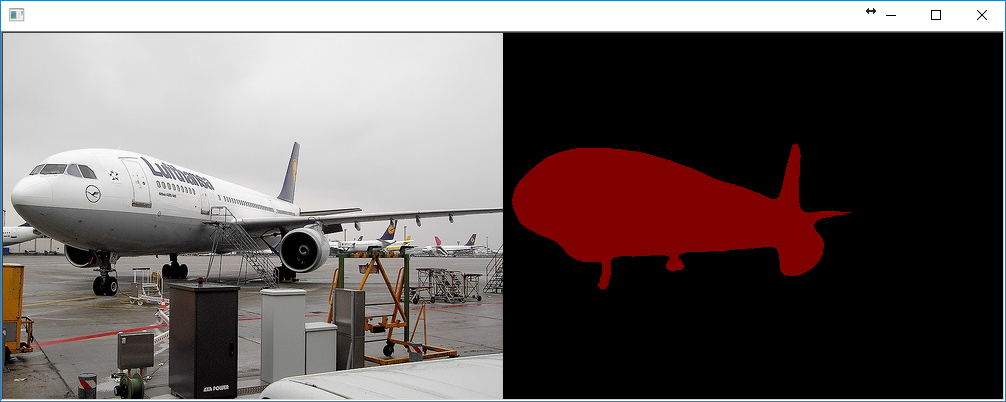## Thursday, December 28, 2017

### A Global Optimization Algorithm Worth Using

Here is a common problem: you have some machine learning algorithm you want to use but it has these damn hyperparameters. These are numbers like weight decay magnitude, Gaussian kernel width, and so forth. The algorithm doesn't set them, instead, it's up to you to determine their values. If you don't set these parameters to "good" values the algorithm doesn't work. So what do you do? Well, here is a list of everything I've seen people do, listed in order of most to least common:
• Guess and Check: Listen to your gut, pick numbers that feel good and see if they work. Keep doing this until you are tired of doing it.
• Grid Search: Ask your computer to try a bunch of values spread evenly over some range.
• Random Search: Ask your computer to try a bunch of values by picking them randomly.
• Bayesian Optimization: Use a tool like MATLAB's bayesopt to automatically pick the best parameters, then find out Bayesian Optimization has more hyperparameters than your machine learning algorithm, get frustrated, and go back to using guess and check or grid search.
• Local Optimization With a Good Initial Guess: This is what MITIE does, it uses the BOBYQA algorithm with a well chosen starting point. Since BOBYQA only finds the nearest local optima the success of this method is heavily dependent on a good starting point. In MITIE's case we know a good starting point, but this isn't a general solution since usually you won't know a good starting point. On the plus side, this kind of method is extremely good at finding a local optima. I'll have more to say on this later.
The vast majority of people just do guess and check. That sucks and there should be something better. We all want some black-box optimization strategy like Bayesian optimization to be useful, but in my experience, if you don't set its hyperparameters to the right values it doesn't work as well as an expert doing guess and check. Everyone I know who has used Bayesian optimization has had the same experience. Ultimately, if I think I can do better hyperparameter selection manually then that's what I'm going to do, and most of my colleagues feel the same way. The end result is that I don't use automated hyperparameter selection tools most of the time, and that bums me out. I badly want a parameter-free global optimizer that I can trust to do hyperparameter selection.

So I was very excited when I encountered the paper Global optimization of Lipschitz functions by Cédric Malherbe and Nicolas Vayatis in this year's international conference on machine learning. In this paper, they propose a very simple parameter-free and provably correct method for finding the $x \in \mathbb{R}^d$ that maximizes a function, $f(x)$, even if $f(x)$ has many local maxima. The key idea in their paper is to maintain a piecewise linear upper bound of $f(x)$ and use that to decide which $x$ to evaluate at each step of the optimization. So if you already evaluated the points $x_1, x_2, \cdots, x_t$ then you can define a simple upper bound on $f(x)$ like this:
$\newcommand{\norm}{\left\lVert#1\right\rVert} U(x) = \min_{i=1\dots t} (f(x_i) + k \cdot \norm{x-x_i}_2 )$ Where $k$ is the Lipschitz constant for $f(x)$. Therefore, it is trivially true that $U(x) \geq f(x), \forall x$, by the definition of the Lipschitz constant. The authors go on to suggest a simple algorithm, called LIPO, that picks points at random, checks if the upper bound for the new point is better than the best point seen so far, and if so selects it as the next point to evaluate. For example, the figure below shows a plot of a simple $f(x)$ in red with a plot of its associated upper bound $U(x)$ in green. In this case $U(x)$ is defined by 4 points, indicated here with little black squares.It shouldn't take a lot of imagination to see how the upper bound helps you pick good points to evaluate. For instance, if you selected the max upper bound as the next iterate you would already get pretty close to the global maximizer. The authors go on to prove a bunch of nice properties of this method. In particular, they both prove mathematically and show empirically that the method is better than random search in a number of non-trivial situations. This is a fairly strong statement considering how competitive random hyperparameter search turns out to be relative to competing hyperparameter optimization methods. They also compare the method to other algorithms like Bayesian optimization and show that it's competitive.

But you are probably thinking: "Hold on a second, we don't know the value of the Lipschitz constant $k$!". This isn't a big deal since it's easily estimated, for instance, by setting $k$ to the largest observed slope of $f(x)$ before each iteration. That's equivalent to solving the following easy problem:
\begin{align}
\text{s.t.} & \quad U(x_i) \geq f(x_i), \quad \forall i \in [1\dots t] \\
\end{align} Malherbe et al. test a variant of this $k$ estimation approach and show it works well.

This is great. I love this paper. It's proposing a global optimization method called LIPO that is both parameter free and provably better than random search. It's also really simple. Reading this paper gives you one of those "duah" moments where you wonder why you didn't think of this a long time ago. That's the mark of a great paper. So obviously I was going to add some kind of LIPO algorithm to dlib, which I did in the recent dlib v19.8 release.

However, if you want to use LIPO in practice there are some issues that need to be addressed. The rest of this blog post discusses these issues and how the dlib implementation addresses them. First, if $f(x)$ is noisy or discontinuous even a little it's not going to work reliably since $k$ will be infinity. This happens in real world situations all the time. For instance, evaluating a binary classifier against the 0-1 loss gives you an objective function with little discontinuities anywhere samples switch their predicted class. You could cross your fingers and run LIPO anyway, but you run the very real risk of two $x$ samples closely straddling a discontinuity and causing the estimated $k$ to explode. Second, not all hyperparameters are equally important, some hardly matter while small changes in others drastically affect the output of $f(x)$. So it would be nice if each hyperparameter got its own $k$. You can address these problems by defining the upper bound $U(x)$ as follows:
$U(x) = \min_{i=1\dots t} \left[ f(x_i) + \sqrt{\sigma_i +(x-x_i)^\intercal K (x-x_i)} \ \right]$ Now each sample from $f(x)$ has its own noise term, $\sigma_i$, which should be 0 most of the time unless $x_i$ is really close to a discontinuity or there is some stochasticity. Here, $K$ is a diagonal matrix that contains our "per hyperparameter Lipschitz $k$ terms". With this formulation, setting each $\sigma$ to 0 and $K=k^2I$ gives the same $U(x)$ as suggested by Malherbe et al., but if we let them take more general values we can deal with the above mentioned problems.

Just like before, we can find the parameters of $U(x)$ by solving an optimization problem:
\begin{align}
\min_{K,\sigma} & \quad \norm{K}^2_F + 10^6 \sum_{i=1}^t {\sigma_i^2} &\\
\text{s.t.} & \quad U(x_i) \geq f(x_i), & \quad \forall i \in [1\dots t] \\
& \quad \sigma_i \geq 0 & \quad \forall i \in [1\dots t] \\
& \quad K_{i,j} \geq 0 & \quad \forall i,j \in [1\dots d] \\
& \quad \text{K is a diagonal matrix}
\end{align} The $10^6$ penalty on $\sigma^2$ causes most $\sigma$ terms to be exactly 0. The behavior of the whole algorithm is insensitive to the particular penalty value used here, so long as it's reasonably large the $\sigma$ values will be 0 most of the time while still preventing $k$ from becoming infinite, which is the behavior we want. It's also possible to rewrite this as a big quadratic programming problem and solve it with a dual coordinate descent method. I'm not going into the details here. It's all in the dlib code for those really interested. The TL;DR is that it turns out to be easy to solve using well known methods and it fixes the infinite $k$ problem.

The final issue that needs to be addressed is LIPO's terrible convergence in the area of a local maximizer. So while it's true that LIPO is great at getting onto the tallest peak of $f(x)$, once you are there it does not make very rapid progress towards the optimal location (i.e. the very top of the peak). This is a problem shared by many derivative free optimization algorithms, including MATLAB's Bayesian optimization tool. Fortunately, not all methods have this limitation. In particular, the late and great Michael J. D. Powell wrote a series of papers on how to apply classic trust region methods to derivative free optimization. These methods fit a quadratic surface around the best point seen so far and then take the next iterate to be the maximizer of that quadratic surface within some distance of the current best point. So we "trust" this local quadratic model to be accurate within some small region around the best point, hence the name "trust region". The BOBYQA method I mentioned above is one of these methods and it has excellent convergence to the nearest local optima, easily finding local optima to full floating point precision in a very small number of steps.

We can fix LIPO's convergence problem by combining these two methods, LIPO will explore $f(x)$ and quickly find a point on the biggest peak. Then a Powell style trust region method can efficiently find the exact maximizer of that peak. The simplest way to combine these two things is to alternate between them, which is what dlib does. On even iterations we pick the next $x$ according to our upper bound while on odd iterations we pick the next $x$ according to the trust region model. I've also used a slightly different version of LIPO that I'm calling MaxLIPO. Recall that Malherbe et al. suggest selecting any point with an upper bound larger than the current best objective value. However, I've found that selecting the maximum upper bounding point on each iteration is slightly better. This alternative version, MaxLIPO, is therefore what dlib uses. You can see this hybrid of MaxLIPO and a trust region method in action in the following video:

In the video, the red line is the function to be optimized and we are looking for the maximum point. Every time the algorithm samples a point from the function we note it with a little box. The state of the solver is determined by the global upper bound $U(x)$ and the local quadratic model used by the trust region method. Therefore, we draw the upper bounding model as well as the current local quadratic model so you can see how they evolve as the optimization proceeds. We also note the location of the best point seen so far by a little vertical line.

You can see that the optimizer is alternating between picking the maximum upper bounding point and the maximum point according to the quadratic model. As the optimization proceeds, the upper bound becomes progressively more accurate, helping to find the best peak to investigate, while the quadratic model quickly finds a high precision maximizer on whatever peak it currently rests. These two things together allow the optimizer to find the true global maximizer to high precision (within $\pm{10^{-9}}$ in this case) by the time the video concludes.The Holder Table Test Function from https://en.wikipedia.org/wiki/File:Holder_table_function.pdf

Now let's do an experiment to see how this hybrid of MaxLIPO and Powell's trust region method (TR) compares to MATLAB's Bayesian optimization tool with its default settings. I ran both algorithms on the Holder table test function 100 times and plotted the average error with one standard deviation error bars. So the plot below shows $f(x^\star)-f(x_i)$, the difference between the true global optimum and the best solution found so far, as a function of the number of calls to $f(x)$. You can see that MATLAB's BayesOpt stalls out at an accuracy of about $\pm{10^{-3}}$ while our hybrid method (MaxLIPO+TR, the new method in dlib) quickly approaches full floating point precision of around $\pm{10^{-17}}$.I also reran some of the tests from Figure 5 of the LIPO paper. The results are shown in the table below. In these experiments I compared the performance of LIPO with and without the trust region solver (LIPO+TR and LIPO). Additionally, to verify that LIPO is better than pure random search I tested a version of the algorithm that alternates between pure random search and the trust region solver (PRS+TR) rather than alternating between a LIPO method and a trust region solver (LIPO+TR and MaxLIPO+TR). Pure random search (PRS) is also included for reference. Finally, the new algorithm implemented in dlib, MaxLIPO+TR, is included as well. In each test I ran the algorithm 1000 times and recorded the mean and standard deviation of the number of calls to $f(x)$ required to reach a particular solution accuracy. For instance, $\epsilon=0.01$ means that $f(x^\star)-f(x_i) \leq 0.01$, while "target 99%" uses the "target" metric from Malherbe's paper, which for most tests corresponds to an $\epsilon > 0.1$. Tests that took too long to execute are noted with a - symbol.

The key points to notice about these results are that the addition of a trust region method allows LIPO to reach much higher solution accuracy. It also makes the algorithm run faster. Recall that LIPO works internally by using random search of $U(x)$. Therefore, the number of calls LIPO makes to $U(x)$ is at least as many as PRS would require when searching $f(x)$. So for smaller $\epsilon$ it becomes very expensive to execute LIPO. For instance, I wasn't able to get results for LIPO, by itself, at accuracies better than $0.1$ on any of the test problems since it took too long to execute. However, with a trust region method the combined algorithm can easily achieve high precision solutions. The other significant detail is that, for tests with many local optima, all methods combining LIPO with TR are much better than PRS+TR. This is most striking on ComplexHolder, which is a version of the HolderTable test function with additional high frequency sinusoidal noise that significantly increases the number of local optima. On ComplexHolder, LIPO based methods require about an order of magnitude fewer calls to $f(x)$ than PRS+TR, further justifying the claims by Malherbe et al. of the superiority of LIPO relative to pure random search.The new method in dlib, MaxLIPO+TR, fares the best in all my tests. What is remarkable about this method is its simplicity. In particular, MaxLIPO+TR doesn't have any hyperparameters, making it very easy to use. I've been using it for a while now for hyperparameter optimization and have been very pleased. It's the first black-box hyperparameter optimization algorithm I've had enough confidence in to use on real problems.

Finally, here is an example of how you can use this new optimizer from Python:
def holder_table(x0,x1):
return -abs(sin(x0)*cos(x1)*exp(abs(1-sqrt(x0*x0+x1*x1)/pi)))

x,y = dlib.find_min_global(holder_table,
[-10,-10],  # Lower bound constraints on x0 and x1 respectively
[10,10],    # Upper bound constraints on x0 and x1 respectively
80)         # The number of times find_min_global() will call holder_table()


Or in C++11:
auto holder_table = [](double x0, double x1) {return -abs(sin(x0)*cos(x1)*exp(abs(1-sqrt(x0*x0+x1*x1)/pi)));};

// obtain result.x and result.y
auto result = find_min_global(holder_table,
{-10,-10}, // lower bounds
{10,10}, // upper bounds
max_function_calls(80));

Both of these methods find holder_table's global optima to about 12 digits of precision in about 0.1 seconds. The C++ API exposes a wide range of ways to call the solver, including optimizing multiple functions at a time and adding integer constraints. See the documentation for full details.

## Wednesday, December 20, 2017

### Dlib 19.8 is Out

Dlib 19.8 is officially out. There are a lot of changes, but the two most interesting ones are probably the new global optimizer and semantic segmentation examples.  The global optimizer is definitely my favorite as it allows you to easily find the optimal hyperparameters for machine learning algorithms.  It also has a very convenient syntax.  For example, consider the Holder table test function:From https://en.wikipedia.org/wiki/File:Holder_table_function.pdf
Here is how you could use dlib's new optimizer from Python to optimize the difficult Holder table function:
def holder_table(x0,x1):
return -abs(sin(x0)*cos(x1)*exp(abs(1-sqrt(x0*x0+x1*x1)/pi)))

x,y = dlib.find_min_global(holder_table,
[-10,-10],  # Lower bound constraints on x0 and x1 respectively
[10,10],    # Upper bound constraints on x0 and x1 respectively
80)         # The number of times find_min_global() will call holder_table()


Or in C++:
auto holder_table = [](double x0, double x1) {return -abs(sin(x0)*cos(x1)*exp(abs(1-sqrt(x0*x0+x1*x1)/pi)));};

// obtain result.x and result.y
auto result = find_min_global(holder_table,
{-10,-10}, // lower bounds
{10,10}, // upper bounds
max_function_calls(80));

Both of these methods find holder_table's global optima to about 12 digits of precision in about 0.1 seconds. The documentation has much more to say about this new tooling.  I'll also make a blog post soon that goes into much more detail on how the method works.

Finally, here are some fun example outputs from the new semantic segmentation example program: